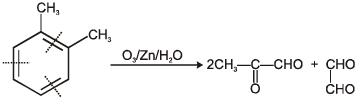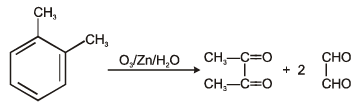Courses

# MCQ (Practice) - Hydrocarbons (Level 2)

## 25 Questions MCQ Test | MCQ (Practice) - Hydrocarbons (Level 2)

Description
This mock test of MCQ (Practice) - Hydrocarbons (Level 2) for Class 11 helps you for every Class 11 entrance exam. This contains 25 Multiple Choice Questions for Class 11 MCQ (Practice) - Hydrocarbons (Level 2) (mcq) to study with solutions a complete question bank. The solved questions answers in this MCQ (Practice) - Hydrocarbons (Level 2) quiz give you a good mix of easy questions and tough questions. Class 11 students definitely take this MCQ (Practice) - Hydrocarbons (Level 2) exercise for a better result in the exam. You can find other MCQ (Practice) - Hydrocarbons (Level 2) extra questions, long questions & short questions for Class 11 on EduRev as well by searching above.
QUESTION: 1

###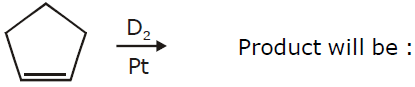Solution: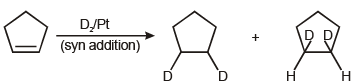QUESTION: 2

### Which of the following reagent not gives syn addition when react with alkyne.

Solution: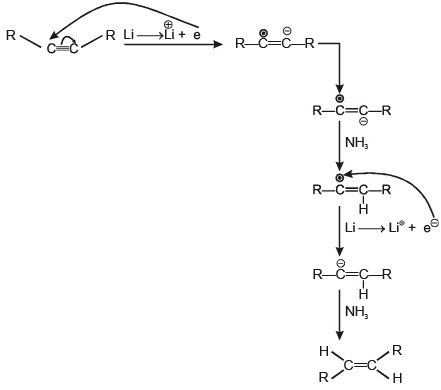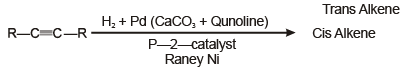QUESTION: 3

###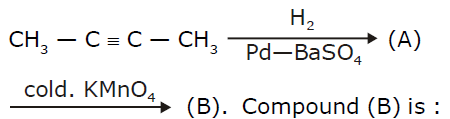Solution: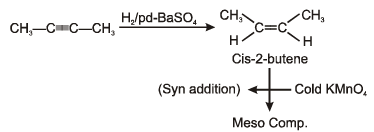QUESTION: 4

Which of the following will decolourise alkaline KMnO4 solution ?

Solution: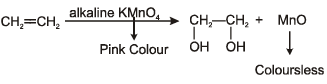QUESTION: 5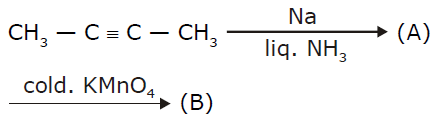Solution: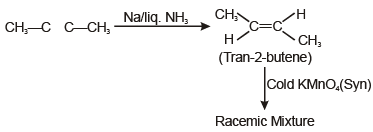QUESTION: 6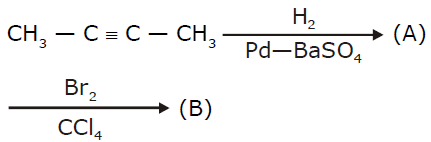Solution: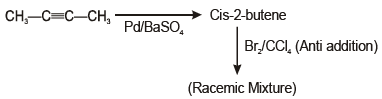QUESTION: 7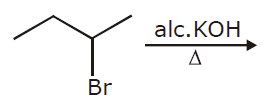Total number of products.

Solution: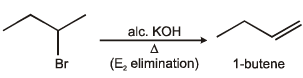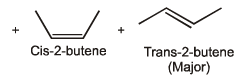QUESTION: 8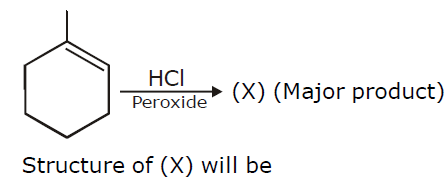Solution: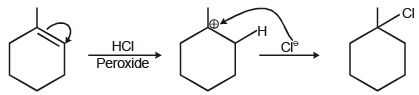QUESTION: 9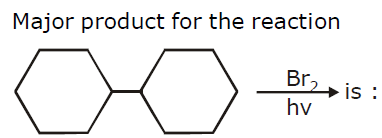Solution: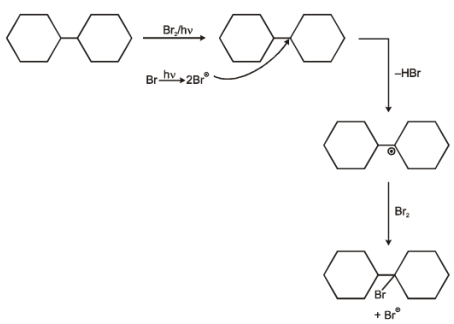QUESTION: 10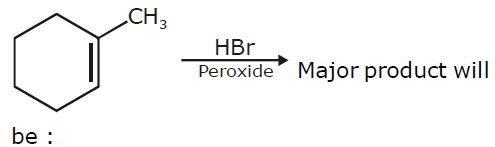Solution: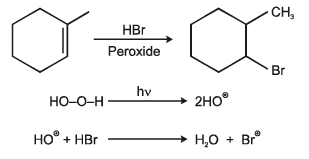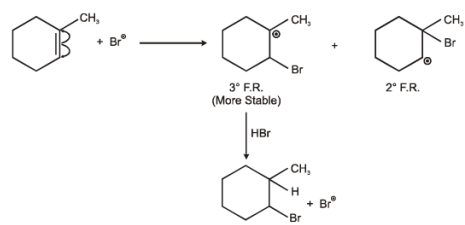QUESTION: 11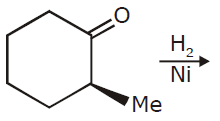Product of above reaction is

Solution: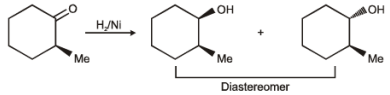QUESTION: 12

Suggest reagent and condition for the asymmetric synthesis of the epoxide given.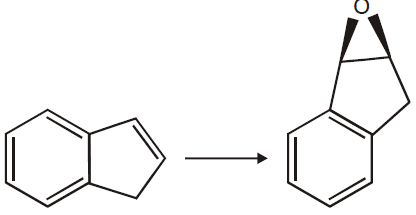Solution:
QUESTION: 13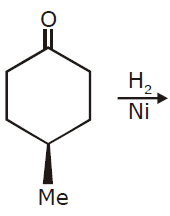product of above reaction is

Solution: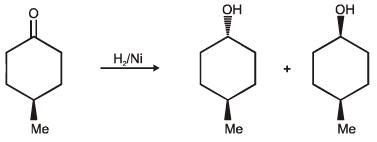QUESTION: 14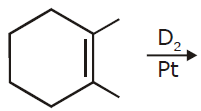product of above reaction is

Solution: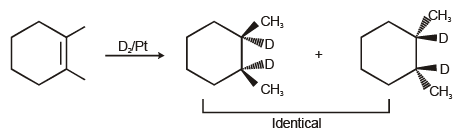QUESTION: 15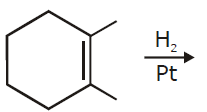product of above reaction is

Solution: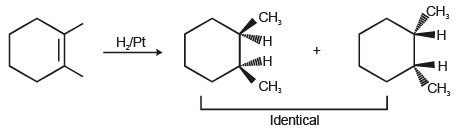QUESTION: 16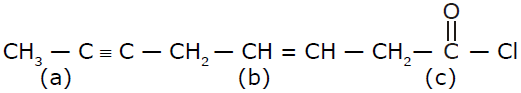Reactivity toward catalytic hydrogenation

Solution: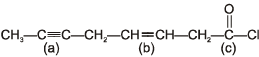more collision probablity having triple bond than double bond. Reactivity order of catalytic hydrogenation C>a>b

QUESTION: 17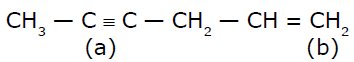Solution: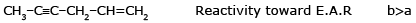QUESTION: 18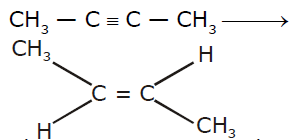Above conversion can be acheived by

Solution: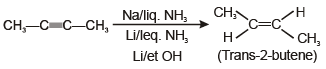QUESTION: 19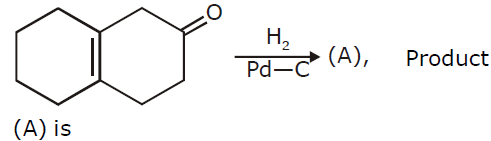Solution: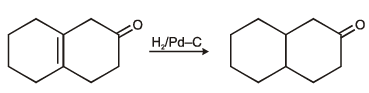QUESTION: 20

Which of following will reacts with Na / liqNH?

Solution: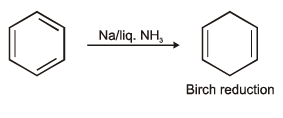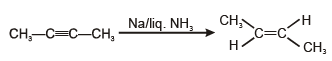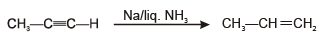QUESTION: 21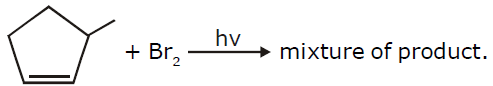Among the following which product will formed minimum amount.

Solution: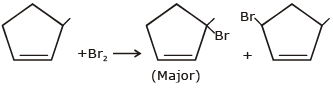QUESTION: 22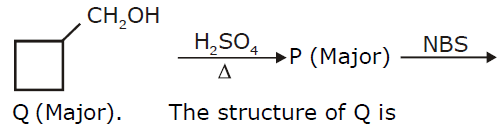Solution: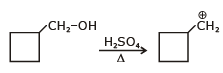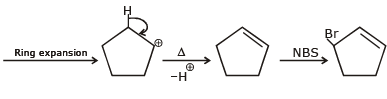QUESTION: 23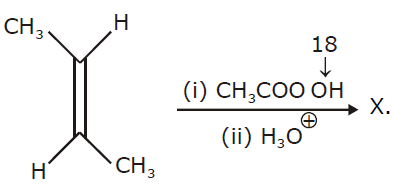The probable structure of ‘X’ is

Solution: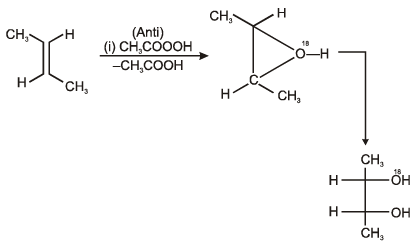QUESTION: 24

Ozonolysis of CH3—CH=C=CH2 will give

Solution: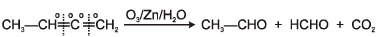QUESTION: 25

O-xylene on ozonolysis will give

Solution: